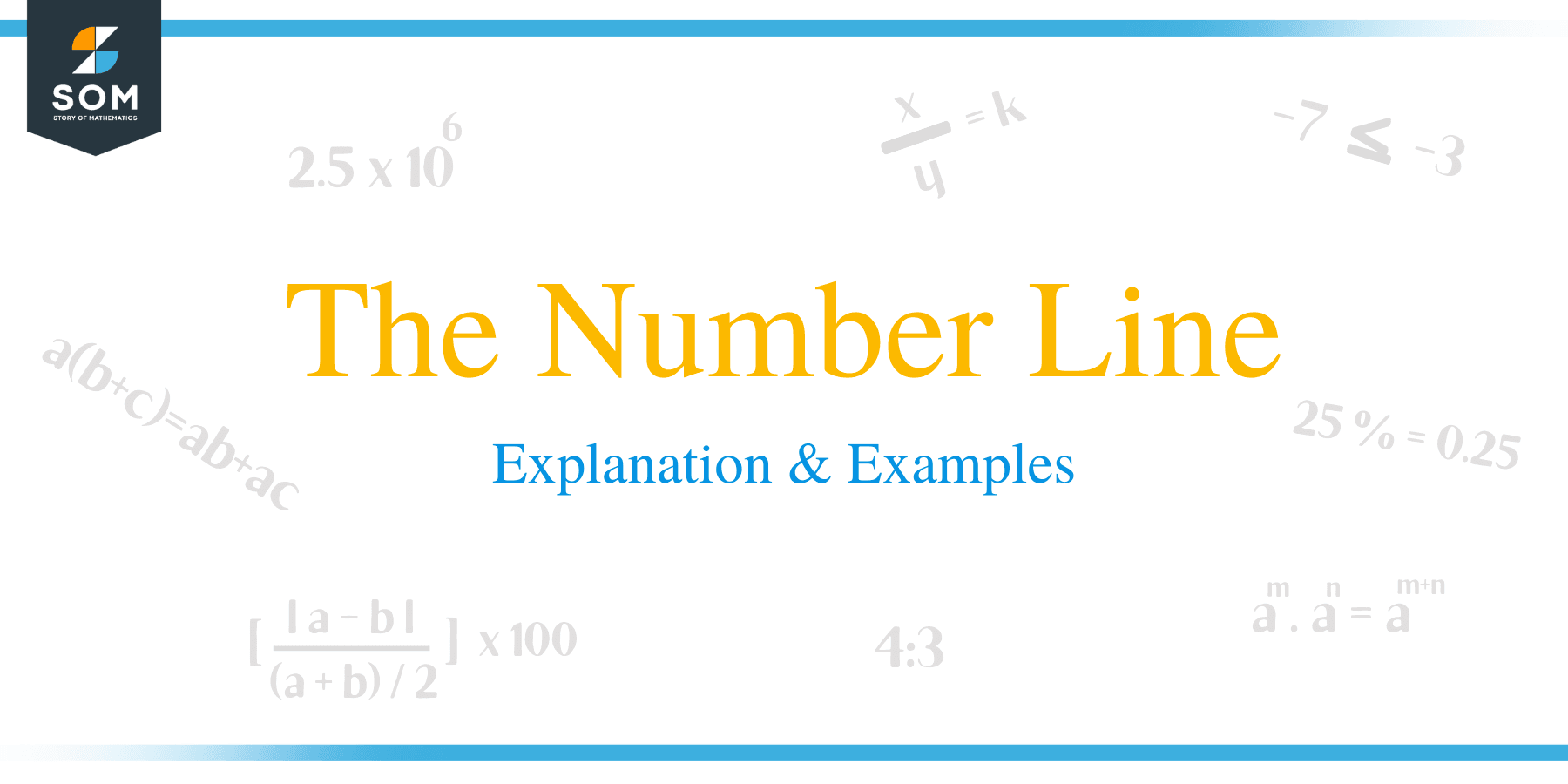# The Number Line – Explanation & Examples

##What is a Number Line?

A number line is defined as the pictorial representation of numbers such as fractions, integers and whole numbers laid out evenly on a straight horizontal line. A number line can be used as a tool for comparing and ordering numbers and also performing operations such as addition and subtraction.

Numbers on a number line include all sets of numbers namely natural and whole numbers. An example of a set of whole numbers is:(0, 1, 2, 3,4,5,6 …….) whereas the natural number include:1, 2, 3, 4, 5, 6….

A number line is composed of three basic parts. In the middle, we the origin usually plotted with zero. You can remember the origin is zero by applying this mnemonic: “The origin of most things is nothing.”

Positive numbers are plotted to the right of the origin while negative numbers are plotted to the left of the origin. Arrows are put on the ends of the horizontal line to show that the line continues to infinity.

To come up with a number line:

• Draw a straight horizontal line of some suitable length.
• Mark points of equal interval.
• Plot the middle point with zero.
• On the right of the origin, plot the marked points starting from +1, +2, +3…… etc.
• Do the same procedure to the left of the origin by plotting numbers starting from -1, -2, -3…… etc.Number lines can be used to teach children how to do addition and subtraction of simple numbers. This method of teaching is important because, the students are able to visualize the operations and therefore be able to learn and understand quickly.

• How to add positive numbers on a number line

As we already know, when two positive numbers are added, the sum will always be positive. For this reason, when adding positive numbers, the direction of movement is to the right.

For example, to one and four, (1+4) = 5: First locate number 1 on the number line and move to the right 4 steps. The number that you land after moving 4 steps from 1 is 5. Therefore, 5 is the answer.

Adding two negative numbers results in a negative number. The direction of adding negative numbers is therefore to left side. For example, to add (-2) + (-3).

First locate -2 on the number line and move 3 steps to the left. The next number is -5. Hence, -5 is the answer.

### Subtraction using a number line

• Subtracting positive numbers

When subtracting positive numbers, move to the left with reference to the value of second number.

For example, subtract 6-3; In this case, the second number is 3 and the first number is 6. Locate number 6 on the number line and move 3 steps to left. The next number is 3 which is the answer.

• Subtracting negative numbers

To subtract two numbers on a number line, move to the right with reference to the value of the second number.

For example, subtract -2 – (-4);

Locate the number -2 on the number line. Move 4 steps to the right which gives 2.

Multiplication can also be done using a number line. In this case, you have to draw a longer number to accommodate the values. You can draw a number line by choosing a suitable scale depending on the numbers being multiplied.For example, to multiply 5 by 3;

You can solve this problem by starting to count from the origin moving 5 steps, three times.

15, in this case, is the correct answer.

### Practice Questions

1. True or False: Zero is the smallest integer.

2. True or False: $17$ is the numerical opposite of $-17$.

3. True or False: The value of negative numbers on a number line increases to the left.

4. True or False: All integers are whole numbers.

5. True or False: All whole numbers are integers.

6. True or False: Zero is neither positive nor negative and it is not an integer.